# Smart pH Sensor

This pH Sensor reads the pH value of any liquid and alerts by SMS with the liquid and its pH value

IntermediateProtip4 hours4,301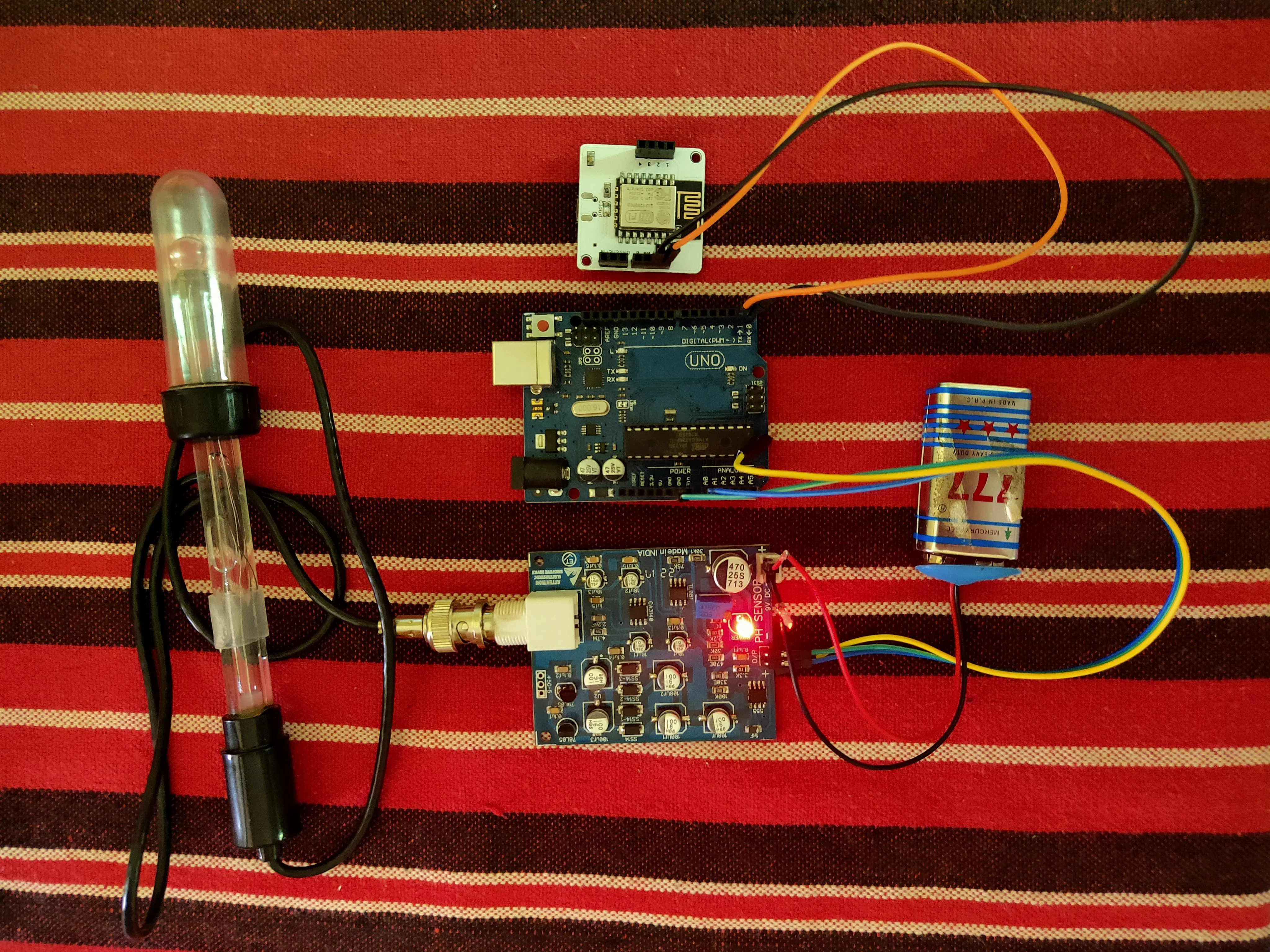## Things used in this project

### Hardware components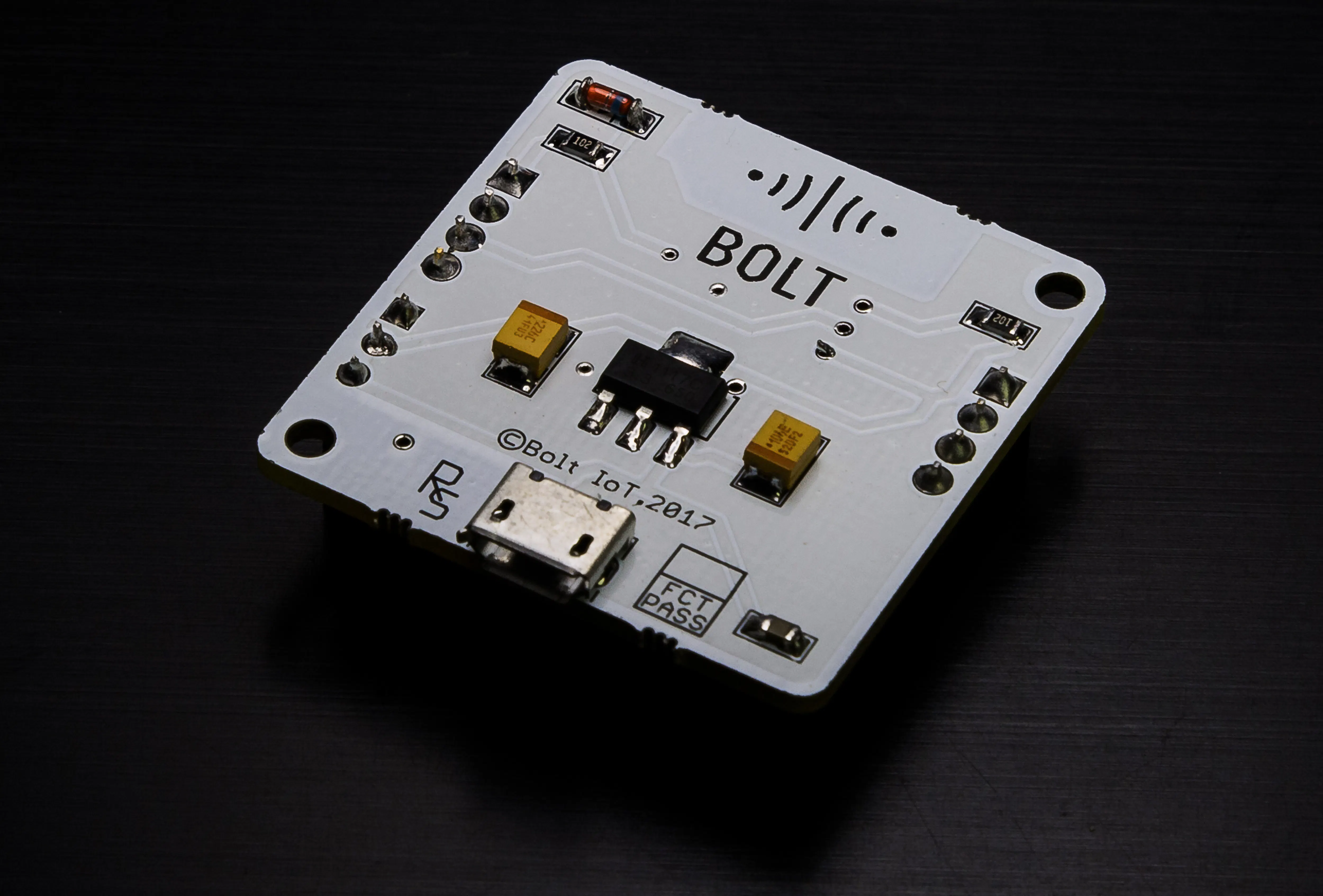Bolt IoT Bolt WiFi Module
×1Arduino UNO
×1
 pH Sensor
×1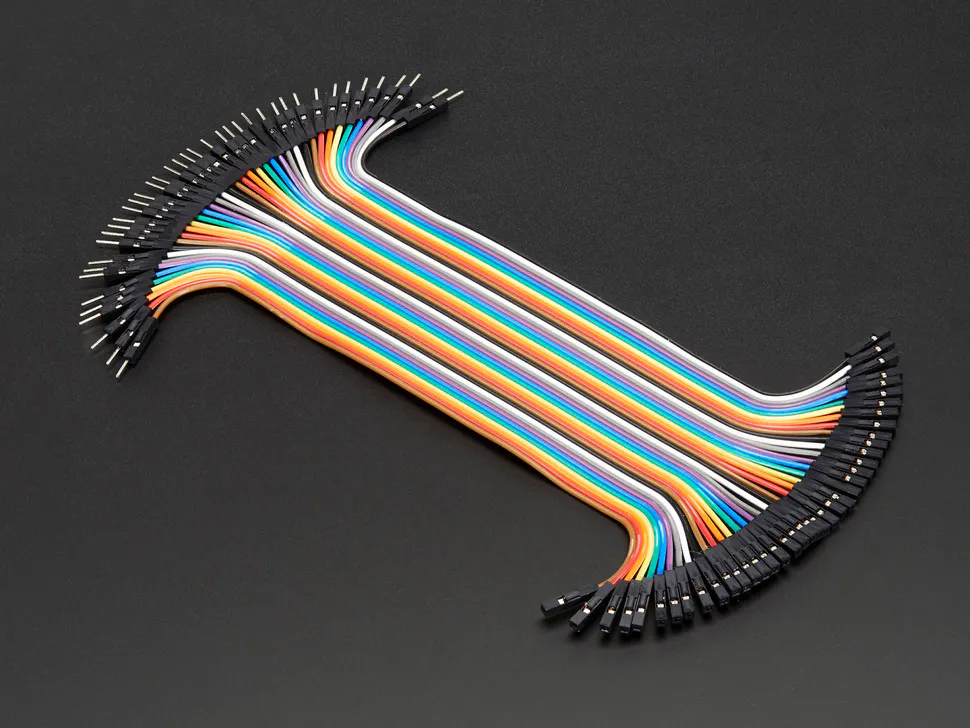Male/Female Jumper Wires
×1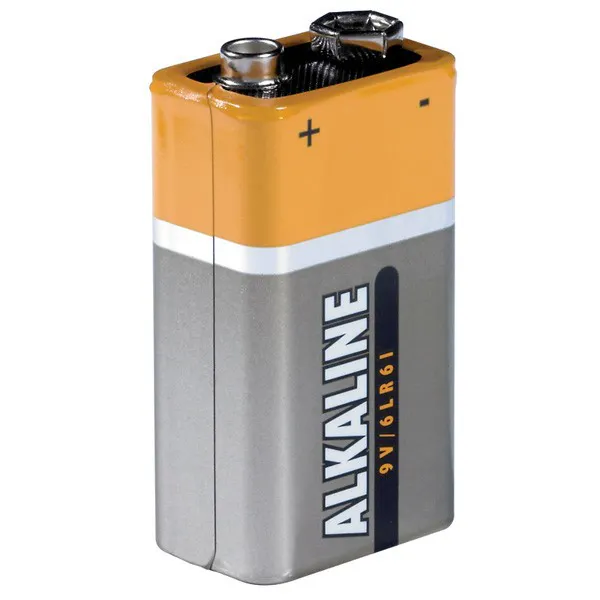9V battery (generic)
×19V Battery Clip
×1

### Software apps and online servicesBolt IoT Bolt Cloud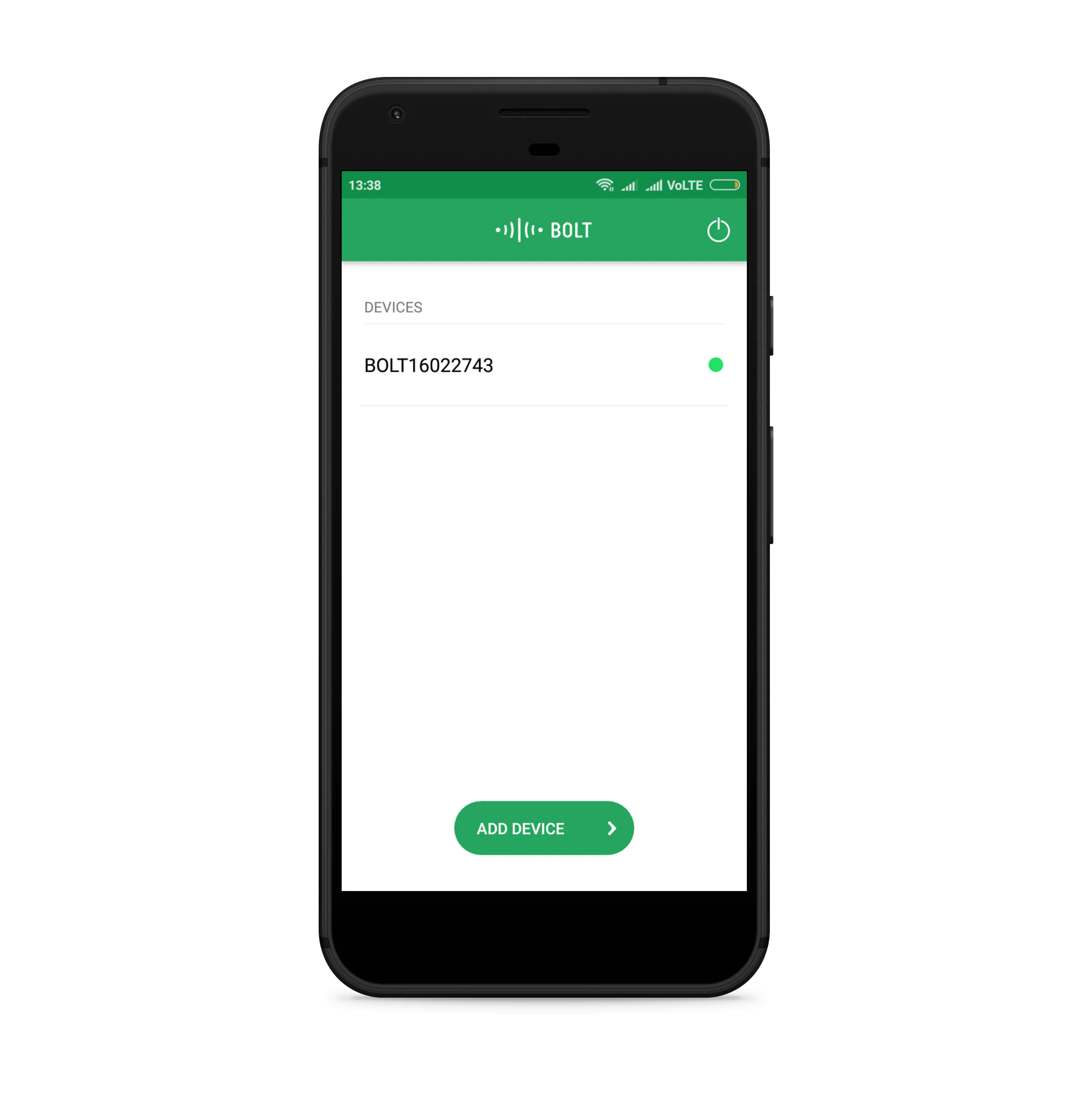Bolt IoT Android AppArduino IDETwilio SMS Messaging API

## Schematics

### pH Sensor Circuit connection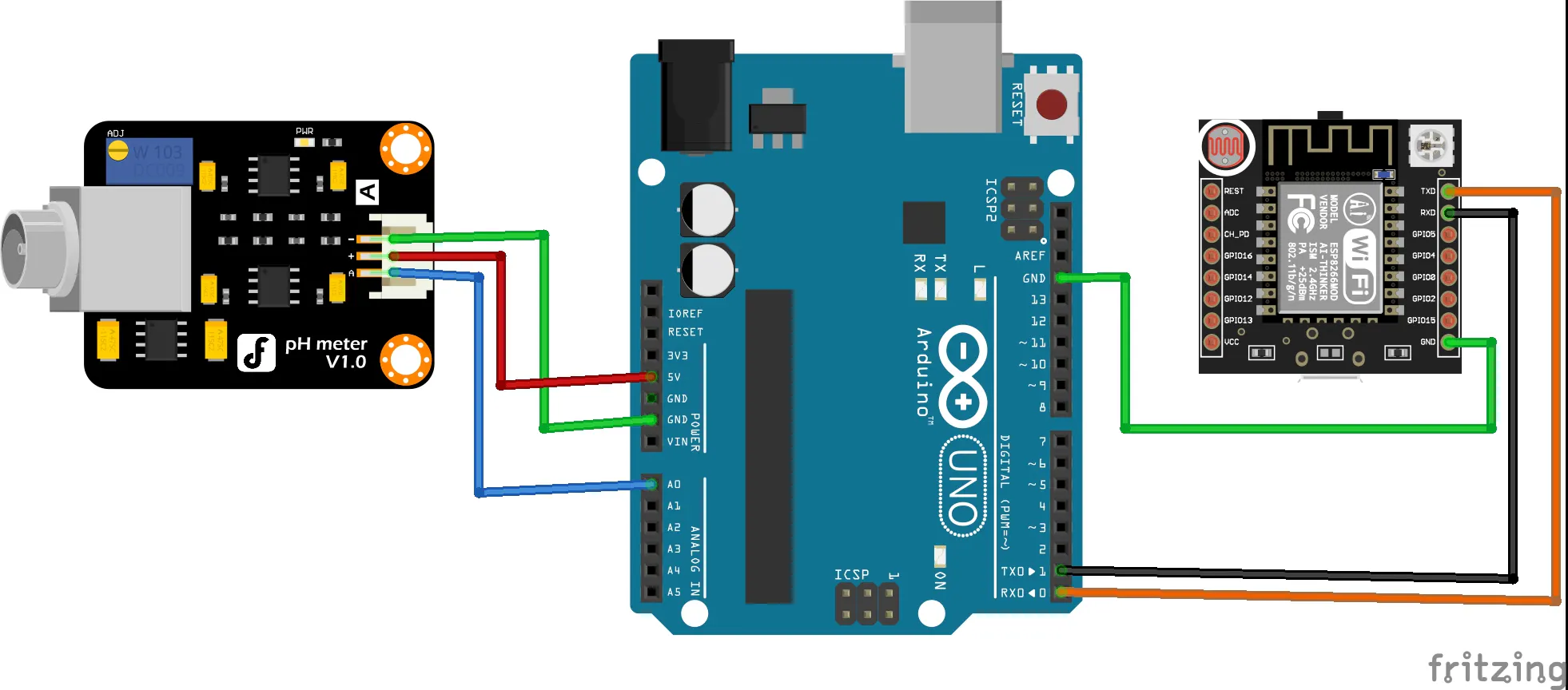## Code

### Python code

Python
```import json, time, conf
from boltiot import Bolt, Sms #Import Sms and Bolt class from boltiot library

value1 = 0
value2 = 6.5
value3 = 7.5
value4 = 14

mybolt = Bolt(conf.API_KEY, conf.DEVICE_ID) #Create object to fetch data
sms = Sms(conf.SID, conf.AUTH_TOKEN, conf.TO_NUMBER, conf.FROM_NUMBER) #Create object to send SMS
print response

while True:
response = mybolt.serialRead('10')  #Fetching the value from Arduino
ph_value = data['value'].rstrip()
print("pH Valueis:"+str(data['value']))
try:
if ph_value > value1 and ph_value < value2:
print("Making request to send SMS")
response = sms.send_sms("The liquid is ACIDIC. pH value of the liquid is:"+str(ph_value))
print("Response recieved:"+ste(response.status))
elif ph_value > value2 and ph_value < value3:
print("Making request to send SMS")
response = sms.send_sms("The liquid is NEUTRAL. pH value of the liquid is:"+str(ph_value))
print("Response recieved:"+ste(response.status))
elif ph_value > value3 and ph_value < value4:
print("Making request to send SMS")
response = sms.send_sms("The liquid is ALKALINE. pH value of the liquid is:"+str(ph_value))
print("Response recieved:"+ste(response.status))
except Exception as e:
print("Error occured: Below are the details")
print(e)
time.sleep(100) #reads sensor value for every 100 seconds
```

### javascript code

JavaScript
```setChartLibrary('google-chart');
setChartTitle('pH Sensor');
setChartType('lineGraph');
setAxisName('Time Stamp','pH Value');
plotChart('time_stamp','value');
```

### Configuration code

Python
```SID = ' '
AUTH_TOKEN = ' '
FROM_NUMBER = ' '
TO_NUMBER = ' '
API_KEY = ' '
DEVICE_ID = ' '
```

### Arduino code

Arduino
```const int analogInPin = A0;
int sensorValue = 0;
unsigned long int avgValue;
float b;
int buf,temp;
void setup() {
Serial.begin(9600);
}

void loop() {
for(int i=0;i<10;i++)
{
delay(100);
}
for(int i=0;i<9;i++)
{
for(int j=i+1;j<10;j++)
{
if(buf[i]>buf[j])
{
temp=buf[i];
buf[i]=buf[j];
buf[j]=temp;
}
}
}
avgValue=0;
for(int i=2;i<8;i++)
avgValue+=buf[i];
float pHVol=(float)avgValue*5.0/1024/6;
float phValue = -5.70 * pHVol + 8.65;
Serial.print("pH Value = ");
Serial.println(phValue);

delay(20);
}
```

## Credits

### Shreyas B V

4 projects • 2 followers

### PavanKumar E

1 project • 0 followers